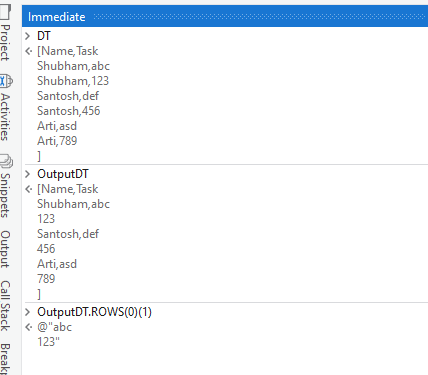# I want solution of my issue

i have one excel sheet (name, task ,coment ) and in name coloumn repeated names are their and i want marge that same name record with one name

1 Like

Do you mean you would want to remove repeated names /String values in that column ?

Could you maybe give an Example ? Are the names separated by Comma ? More details would help us give you better suggestions.

Thanks for reaching out to community.

We need to use “Remove Duplicates” activity here.

Here are the steps you can follow.

Use the “Excel Application Scope” activity to open the Excel file.
Use the “Read Range” activity to read the data from the Excel sheet into a DataTable variable.
Use the “Remove Duplicates” activity and specify the column name (“Name”) that you want to remove duplicates from.
Save the updated DataTable using the “Write Range” activity.
Close the Excel file using the “Excel Application Scope” activity.

This will help you. Happy AutomationRegards,

1 Like

but other data will merge or not

It doesnt merge any other data. So if you have duplicate rows in your Excel sheet, the activity will remove them and keep only one row with unique values in the specified columns.

1 Like

To merge the same name records in an Excel sheet using UiPath, you can follow these steps:

1. Read the Excel sheet using the Read Range activity and store the output in a DataTable variable.
2. Use the Group By function to group the records based on the name column. This will create a new DataTable with unique name records.
``````groupByResult = From row In dt.AsEnumerable()
Group row By ColumnName = row("ColumnName") Into Group
Select New With {
.ColumnName = ColumnName,
.Count = Group.Count(),
.Sum = Group.Sum(Function(row) Decimal.Parse(row("NumericColumnName").ToString()))
}

``````
1. Use a For Each Row activity to iterate through the grouped DataTable.
2. Inside the For Each Row activity, create a new DataRow variable and use the Assign activity to set its values as the corresponding values from the current row of the grouped DataTable.
3. Use the Add Data Row activity to add the new DataRow variable to a new DataTable that will contain the merged records.
4. Write the merged records DataTable to a new Excel sheet using the Write Range activity.

hi @ABHIMANYU_THITE1 please send me snipet or screenshot for the solution

Hi @Shubham_Bidwai, Did you try given above comments? can you share what result you are getting?

Apologies. But could you provide us with an Example, so that we could set an approach towards creating the solution.

We would want to know how Repeated names are present in the Excel Sheet (Input Data)
And we would want to Check how the Merging the same names one name happens (Expected Output )

If screenshots are provided for these, it would be much helpful for us to suggest faster.

Check with the below Steps :

1. Read the Excel Sheet as a Datatable using `Read Range` Activity. Get the Datatable, say `DT`.

2. Next, Create another Datatable to create the Output data, say `OutputDT`. Assign the clone of the Input datatable to this Output Datatable like below using an `Assign` Activity.

``````OutputDT  = DT.Clone
``````
1. Next, we’ll use the below Linq in an `Assign` Activity to Group By and Concatenate the values based on the Column `Name` :
``````OutputDT = (From d In DT.AsEnumerable
Group d By k=d("Name").toString.Trim Into grp=Group
Let cn = String.Join(Environment.NewLine, grp.Select(Function (r) r("Task").toString).toArray)
Let ra = New Object(){k, cn}
``````

Visuals :Let us know if you are able to understand the above approach.

Also take a look at the Grouping options and documentation :

1 Like

First assign:-
`dt_Final = dt_Input.Clone()`

Second assign:-

``````dt_Final = (From x In dt_Input
Group x By k=x("Name").tostring.trim Into grp=Group
Let ma = String.Join(Environment.NewLine, grp.select(Function(y) y("Task").tostring.trim).ToArray())
Hope this may help you